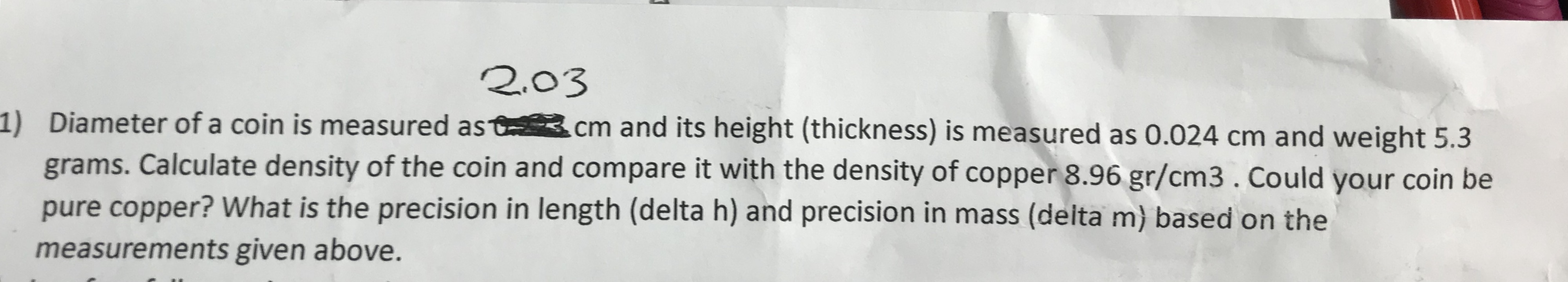# 2.03 ) Diameter of a coin is measured ast.cm and its height (thickness) is measured as 0.024 cm and weight 5.3 grams. Calculate density of the coin and compare it with the density of copper 8.96 gr/cm3. Could your coin be pure copper? What is the precision in length (delta h) and precision in mass (delta m) based on the measurements given above.

Questionhelp_outlineImage Transcriptionclose2.03 ) Diameter of a coin is measured ast.cm and its height (thickness) is measured as 0.024 cm and weight 5.3 grams. Calculate density of the coin and compare it with the density of copper 8.96 gr/cm3. Could your coin be pure copper? What is the precision in length (delta h) and precision in mass (delta m) based on the measurements given above. fullscreen